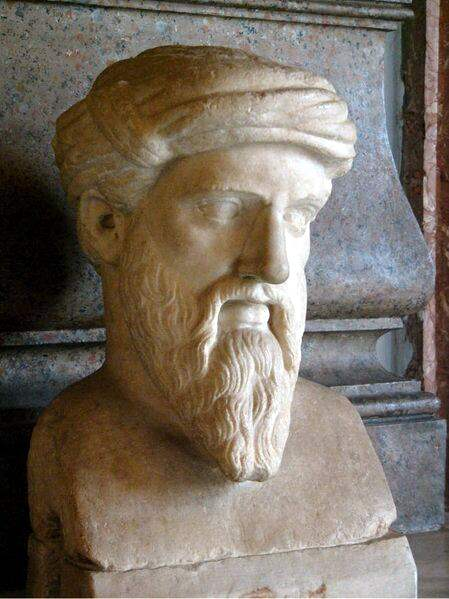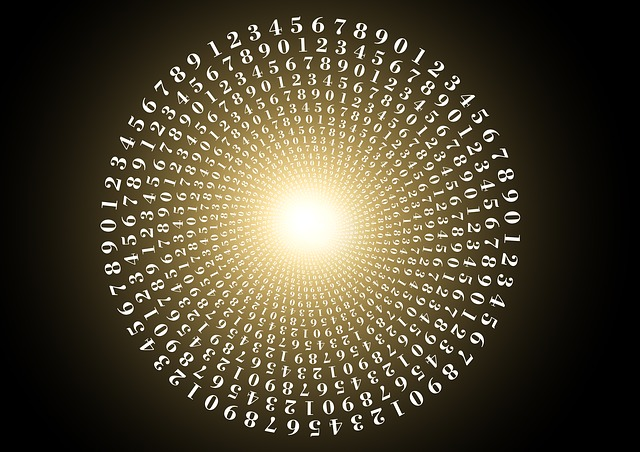## Devil## Wednesday, August 5, 2015

### Numerology, Taking a Look at an Age Old PracticeA bust of Pythagoras; by Galilea at German Wikipedia, GFDL via Wikimedia Commons

## A Numerology Rundown

### What this ancient practice is and how it (basically) works

Numbers, on one level or another, have been a cornerstone of civilization and technological advancement since human beings have walked upright. One early proponent of numerology was Pythagoras, who helped formalize ideas found in the Kabbalah and other ancient texts. He did not study numerology though, he studied isopsephy. As with any discipline that's sincerely old, there is a lot of debate over just who "founded" numerology and who made the greatest contributions. Unfortunately, there aren't a lot of hard and fast answers to be found. Still, we know that Pythagoras opened peoples' minds to using numbers in various ways, and others built upon his concepts.

The foundations of numerology were long established by the early days of the 20th century, when Mrs. L. Dow Balliett and Dr. Juno Jordan worked to refine and mould it into becoming a modern practice; these two individuals are the ones that played perhaps the largest part in defining the methodology of what is today referred to as the Pythagorean system.

### Form and Function

Numerology studies the relationship between numbers and events in one's life. To be clear, numerology is often considered an occult, or metaphysical practice, which means that the aforementioned relationships can't always be accounted for by scientific or practical means. And by the same token, practitioners of numerology are often drawn to its spiritual component, as is commonly the case with occult practices.

Numbers can apply to science in unusual ways; at times a theory may be suggestive of certain logic used in numerology. Such was the case in the discovery of atomic triads, an observation that was originally written off as numerology or pseudo science, that eventually turned out to be correct. In fact, by looking at the numerical relationship between the lightest and heaviest element in an atomic group and averaging those sums to find a third element, Johann Wolfgang Dobereiner was able to lay the groundwork for the periodic table.

However, in its most common applications, numerology is useful to those looking for patterns and guidance in their lives. By examining the interrelation of numbers and the harmonic vibrations associated with them, some people use numerology to make decisions about events as important as weddings and business investments. Other people use numerological principles to structure their daily routines and redefine their goals. One of the most appealing aspects of numerology is that its uses are limited only by the practitioner's creativity.

### Nuts and Bolts

One of the core ideas underpinning numerology is the ancient practice of gematria. Gematria involves assigning numerical value to written characters, particularly in an alphabetical system; however, we will have to settle for a mere mention on that in order to stay on topic.

Pythagorean numerology makes use of eleven numbers: 1-9, and 11 and 22. Larger numbers like 5,345 are read as follows: 5 + 3 + 4 + 5 = 17; then 1 + 7 = 8. Thus even a large number as such can be reduced down to the single digit, 8.

Two of the most important introductory processes in numerology are, first, calculating your life path number, and second, calculating the value of your full name to find, amongst other things, your expression number.### An Example of How to Calculate a Life Path Number

If your birth date involves double digits, add them to arrive at a single digit, unless you have any master numbers, which are not reduced.

If you were born on 4/25/2009 you would first add the digits:

Month: 4
Day: 2 + 5 = 7
Year: 2 + 0 + 0 + 9 = 11

then you'd add the resulting digits together to arrive at a life path number of 22, which incidentally, is another master number. As already stated, normally a master number is not reduced; but if you were not considering the master numbers (either for simplicity or because you didn't want to) you could treat it as you would treat all other double digit numbers by adding its digits together (2 + 2) to arrive at a single digit (4).

### An Example of How to Calculate an Expression, or Destiny Number

The next calculation you make will require the use of your full name at birth, that's first, middle and last; do not use abbreviations.

To calculate the sum of your full name, you will need to know the numbers that correspond to the letters in your name:

1 = a, j, s
2 = b, k, t
3 = c, l, u
4 = d, m, v
5 = e, n, w
6 = f, o, x
7 = g, p, y
8 = h, q, z
9 = i, r

So, if your name were Billy Jon Brown and we were consulting the table above, we could convert your first name to the digits 29337. Then we would add each digit together:

Billy = 2 + 9 + 3 + 3 + 7 = 24; then 2 + 4 = 6
Jon = 1 + 6 + 5 = 12; then 2 + 1 = 3
Brown = 2 + 9 + 6 + 5 + 5 = 27; then 2 + 7 = 9

then we'd take each resulting single numeral and add them together to get 18, which when reduced provides your expression (or destiny) number, which is 9. Note that if the sum of any one of the names is a master number, do not reduce it before adding the sums together.

### The Wrap-up

These are just the very basics of numerology, a sampler, if you will. The calculations and the ways in which they're interpreted can become vastly more subtle and complex, depending upon the experience and confidence of the numerologist. Hopefully this gives you a clear easy intro into a field that is nearly as old as humankind and is intrinsically woven into our collective history.